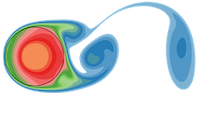FLASH-X Doxygen Generated Documentation From Interface Source Code
Grid_getDomainBoundBox.F90 File Reference

Go to the source code of this file.

## Functions/Subroutines

subroutine Grid_getDomainBoundBox (boundBox)

## ◆ Grid_getDomainBoundBox()

 subroutine Grid_getDomainBoundBox ( real boundBox )

Gets the physical domain bounding box of the entire domain. For each dimension the left (lower or forward) physical coordinate of the domain edge and the right (upper or back) physical coordinate of the domain edge is returned. See arguments below for more detail.

boundBox - returned array holding the boundBox coordinates in each dimension

for readability, in constants.h we define IAXIS = 1, JAXIS = 2, KAXIS = 3

boundBox(1,IAXIS) = left edge coordinate of domain in x direction boundBox(2,IAXIS) = right edge coordinate of domain in x direction boundBox(1,JAXIS) = top edge coordinate of domain in y direction boundBox(2,JAXIS) = bottom edge coordinate of domain in y direction boundBox(1,KAXIS) = front edge coordinate of domain in z direction boundBox(2,KAXIS) = back edge coordinate of domain in z direction

EXAMPLE

In 2 dimensions, if physical coordinates are ...

________________(0.5 1.0) | | | | | | | | | | | | | | |_______________ | (-0.5, 0.0)

boundBox(1, IAXIS) = -0.5 boundBox(2, IAXIS) = 0.5 boundBox(1, JAXIS) = 0.0 boundBox(2, JAXIS) = 1.0

Definition at line 66 of file Grid_getDomainBoundBox.F90.